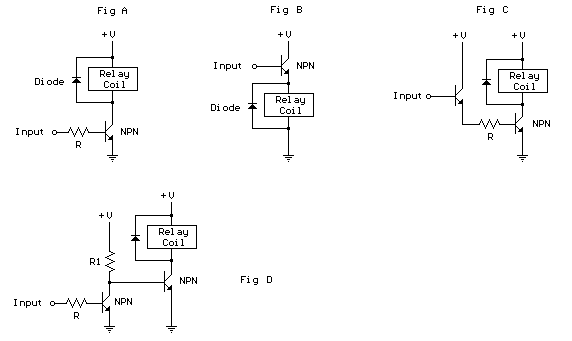### Controlling a relay with a digital logic level.

The schematic below illustrates 4 methods of controlling a relay with a digital logic signal. Figure (A) can probably be used in most cases where the relay coil requires 100 mA or less and the input current is 2 milliamps or more. The resistor value (R) is determined from the input voltage and the available current. For example, a 5 volt input signal supplying 2 milliamps would require (5-.7)/.002 = 2150 ohms, or a 2.2K standard value. If the transistor has a minimum current gain of 50, there will be 100 mA of current available for the relay coil. The following table shows various resistor values that can be used to obtain various relay coil currents assuming a transistor current gain of 50 such as the 2N3053. 74XX refers to standard TTL logic, 74LSXX refers to low power TTL logic, 74HC is high speed CMOS and CD40XX is the older CMOS devices. The currents given are approximate values and may not be correct for all devices but should be close.
```Input Voltage     Input Current     Relay coil current      Standard Resistor

4             74LSXX  .0004       20 milliamps            8.2K
4             74XX    .0008       40 milliamps            4.3K
4             74SXX   .001        50 milliamps            3.3K
5             74HCXX  .004        200 milliamps           1K
6             74HCXX  .004        200 milliamps           1.3K
5             CD40XX  .0003       15 milliamps            13K
12            CD40XX  .0006       30 milliamps            18K
```
Figure B can be used when the input voltage is the same as the relay coil voltage. The voltage on the emitter of the transistor will be about 0.7 volts less than the input, so a 12 volt relay would operate on 11.3 which should be close enough. No resistor is needed since the emitter follower configuration presents a high impedance at the input. The input current will be the relay coil current divided by the transistor gain. For example a 120 ohm relay coil will draw 100 mA at 12 volts and if the transistor gain is 50, the input current will be about 2 milliamps.

Figure C can be used to provide additional gain when the input current is very small. You can also use a Darlington transistor in place of the two transistors which is a better approach, but this idea works just as well when you don't have a Darlington transistor handy. The overall gain will be the product of the individual gains of the two transistors or about 2500 for two transistor with a gain of 50 each. This will enable supplying over 250 mA to the relay with only 100 microamps of input current. The R value will depend on the input voltage and current and gain of the first transistor. For example, using a 5 volt input and 100 microamp current and transistor gain of 50, the R value will be 5 minus two diode drops (5 - 1.4) divided by the input current times 50, or about (5 - 1.4) / (.0001 * 50) = about 750 ohms. So this setup can be used when controlling heavy duty relays with low power CMOS logic signals.

Figure D can be used to reverse the relay action so that it engages when the input is low and disengages when the input is high. The R value is determined the same as in Figure A. The R1 value should be high enough to ensure saturation of the first stage and low enough to saturate the second stage. For example, if a 12 volt relay coil requires 100 mA and the driving transistor gain is 50, then the base current will be 100/50= 2 mA and the R1 value must be less than 6000 ohms so that 2 mA does not drop more than the supply voltage of 12. If the first transistor gain is 50 and the input current is 100 microamps, the collector current will be 5 mA and the R1 value must be greater than 2400 ohms so that 5 mA drops the entire supply voltage of 12. So we need to select something between these two limits of 2.4K to 6K, something around 4.3K would be near the midrange.